## Example Questions

### Example Question #1 : Mode

Find the mode of the following set of numbers:

4,6,12,9,12,90,12,18,12,12,12,4,4,4,9,7,76

12

90

18

6

4

12

Explanation:

Mode is the item that appears most often.

### Example Question #2 : Mode

Find the mode: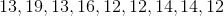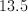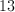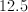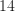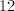Explanation:

The mode is the number that appears most frequently in a given set.

### Example Question #1 : Mode

The Bobcats scored 91, 83, 82, 82, 78, 87, 89, 96, and 86 points in their last nine home games. What is the difference between the average and the mode of their points scored?Cannot be determinedExplanation:

The average is the sum of the points scored in the last nine games divided by 9, which equals 86. The mode is the score which occurs most often, 82. 86 – 82 = 4

### Example Question #4 : Mode

A survey has shown that the number of children that newlyweds plan to have is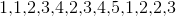respectively. What is the mode of the number of children newlyweds tend to have based on this survey?Explanation:

To solve this problem, we must be clear on the definition of the mode of a set of numbers. The mode is defined as the number that occurs the most frequently within a set of numbers. In this case, we can see that the number 1 occurs 3 times, the number 2 occurs 4 times, the number 3 occurs 3 times, the number 4 occurs 2 times and the number 5 occurs only one time. Therefore the mode of this survey is 2.

### Example Question #5 : Mode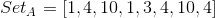Quantity A: The mode of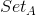Quantity B: The median ofThe relationship cannot be determined.

Quantity A is greater.

The two quantities are equal.

Quantity B is greater.

The two quantities are equal.

Explanation:

Begin by organizing the set in numerical order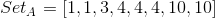The mode is the value which repeats the most, so

Quantity A:Now, since there are an even number of values, the median is the average of the two middle values: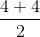Quantity B:The two quantities are equal.

### Example Question #6 : Mode

What is the mode of the following set of numbers?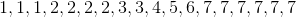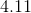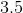The mode is the most occuring value in a set.is the mode because it occurs 6 different times in the set.